# Transportation Problem | Set 7 ( Degeneracy in Transportation Problem )

• Difficulty Level : Hard
• Last Updated : 03 Dec, 2019

This article will discuss degeneracy in transportation problem through an explained example.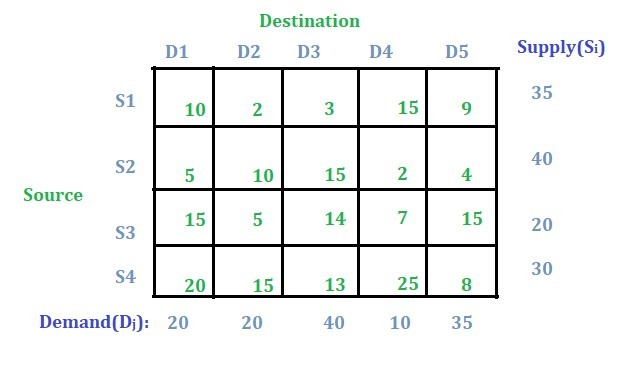Solution:
This problem is balanced transportation problem as total supply is equal to total demand.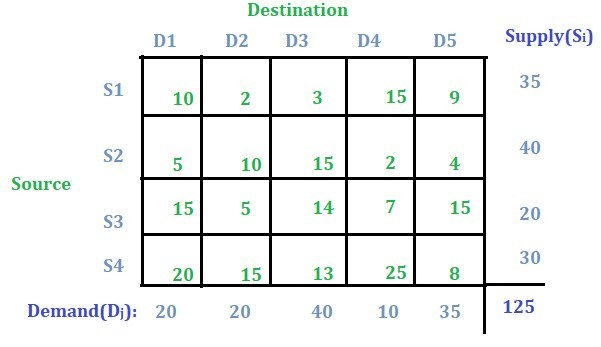Initial basic feasible solution:
Least Cost Cell Method will be used here to find the initial basic feasible solution. One can also use NorthWest Corner Method or Vogel’s Approximation Method to find the initial basic feasible solution.

Using Least Cost Cell Method we get the following solution.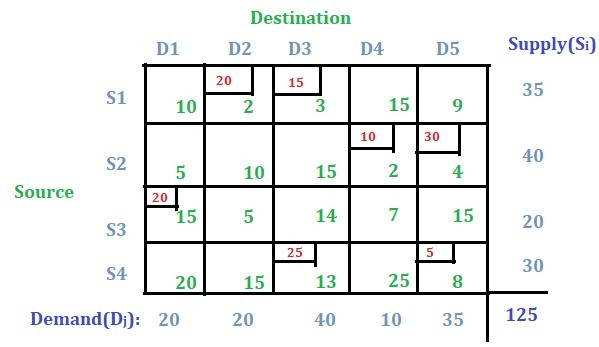Optimization of the solution using U-V Method:
Check whether m + n – 1 = total number of allocated cells. In this case m + n – 1 = 4 + 5 – 1 = 8 where as total number of allocated cells are 7, hence this is the case of degeneracy in transportation problem. So in this case we convert the necessary number (in this case it is m + n – 1 – total number of allocated cells i.e. 8 – 7 = 1) of unallocated cells into allocated cells to satisfy the above condition.

Steps to convert unallocated cells into allocated cells:

• Start from the least value of the unallocated cell.
• Check the loop formation one by one.
• There should be no closed-loop formation.
• Select that loop as a new allocated cell and assign a value ‘e’.

The closed loop can be in any form but all the turning point should be only at allocated cell or at the cell from the loop is started.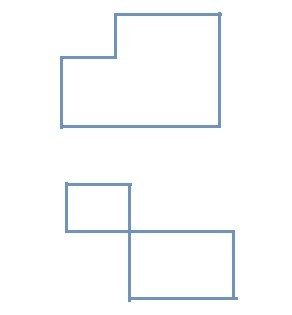There are 13 unallocated cells. Select the least value (i.e. 5 in this case) from unallocated cells. There are two 5s here so you can select randomly any one. Lets select the cell with star marked.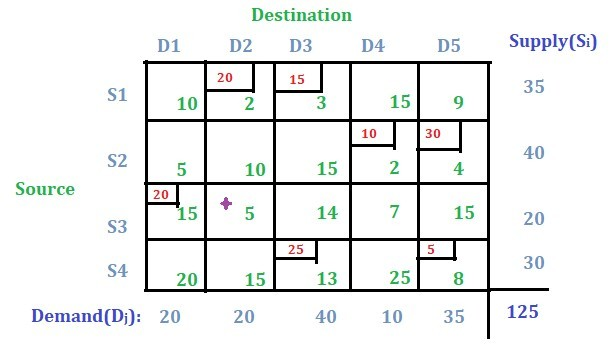Check if there is any closed-loop formation starting from this cell. If a closed-loop is drawn from this cell following the condition for closed-loop then it can be observed that this cell cannot be reached to complete the closed-loop. So this cell will be selected and assigned a random value ‘e’.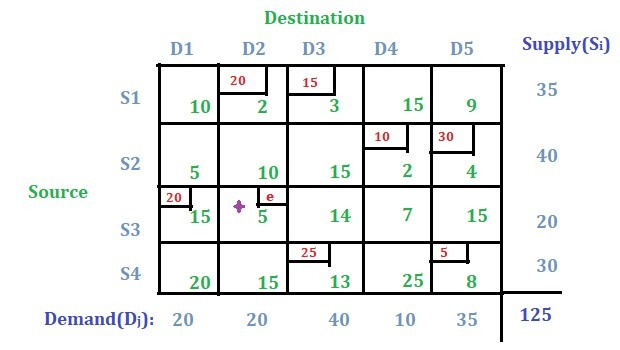Note: If the closed loop would have been formed from that cell then we would try another cell with least value and do the same procedure and check whether closed loop is possible or not.

Now total number of allocated cells becomes 8 and m + n – 1 = 4 + 5 – 1 = 8. Now this solution can be optimized using U-V method. We get the below solution after performing optimization using U-V method.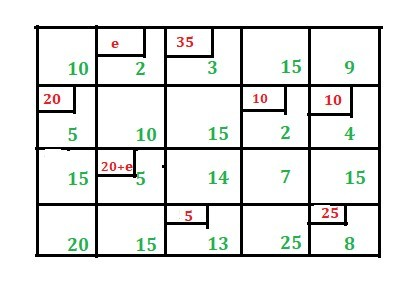The presence of two ‘e’ in the final solution means after doing some iterations during optimization, the condition for degeneracy will be met once again.

While finding the total cost, just leave the ‘e’ and multiply the allocated value with its cell’s cost value and add all of them. So, the transportation cost is (35 * 3) + (20 * 5) + (10 * 2) + (10 * 4) + (20 * 5) + (5 * 13) + (25 * 8) = 630.

My Personal Notes arrow_drop_up